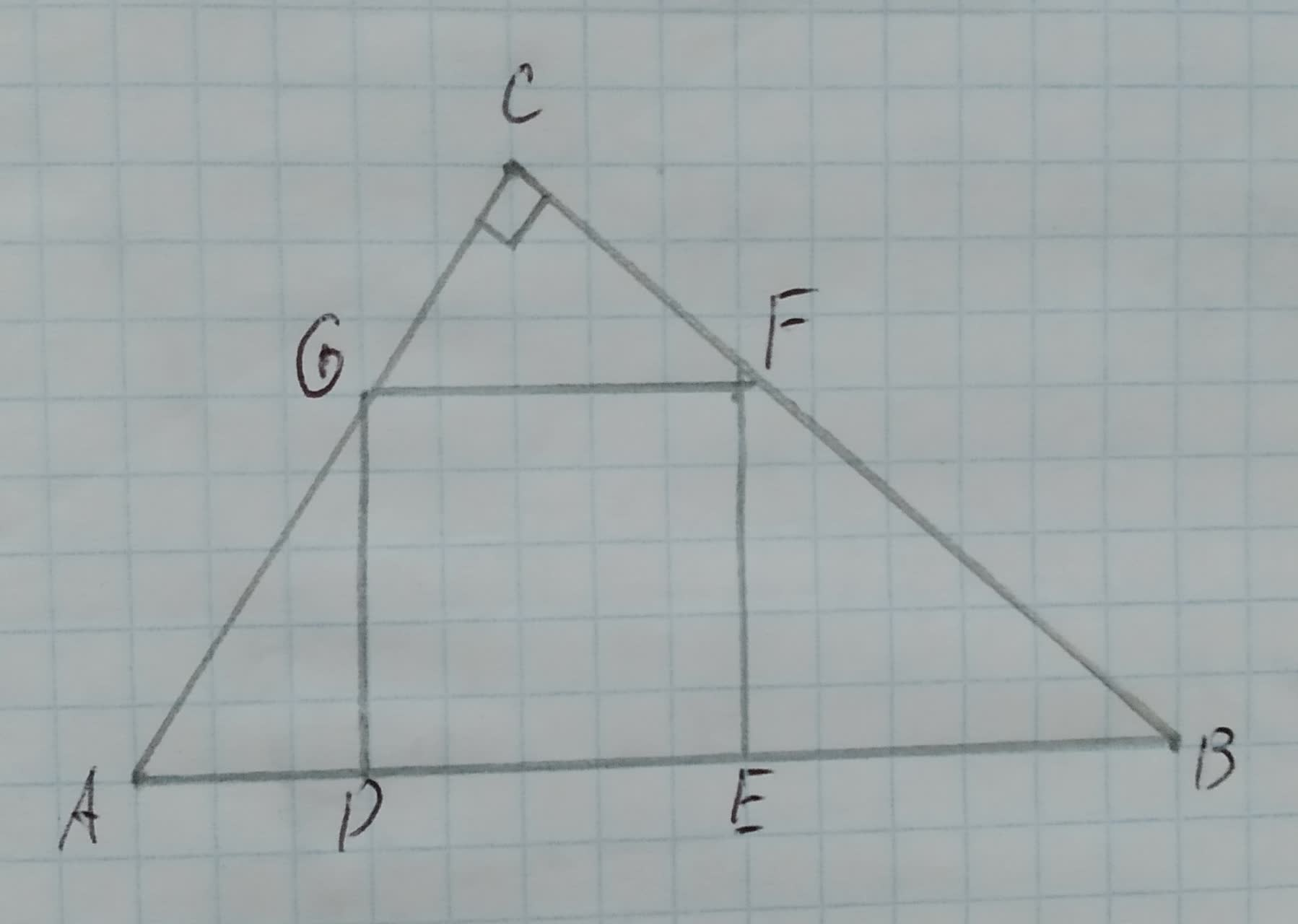# Proving Similarity in the figure DEFG is a square. Prove the following: DE=sqrt(AD*EB) Given: The given figure is, 12210202691.jpgCheyanne Leigh 2020-10-23 Answered
Proving Similarity in the figure DEFG is a square. Prove the following:
$DE=\sqrt{AD\cdot EB}$
Given:
The given figure is,You can still ask an expert for help

• Questions are typically answered in as fast as 30 minutes

Solve your problem for the price of one coffee

• Math expert for every subject
• Pay only if we can solve itBrittany Patton
Approach:
All the sides of a square are equal in length.
Calculation:
It is proved that,
AD * EB = DG * FE(1)
In the figure DEFG is a square, thus, DG and IE can be replaced by DE as they all are sides of a square and equal in length.
Substitute DE for DG and FE in equation (1).
AD * EB = DE * DE
${\left(DE\right)}^{2}=AD\cdot EB$
$DE=\sqrt{AD\cdot EB}$
Therefore, it is proved that $DE=\sqrt{AD\cdot EB}$.
Conclusion:
Hence, it is proved that $DE=\sqrt{AD\cdot EB}$.